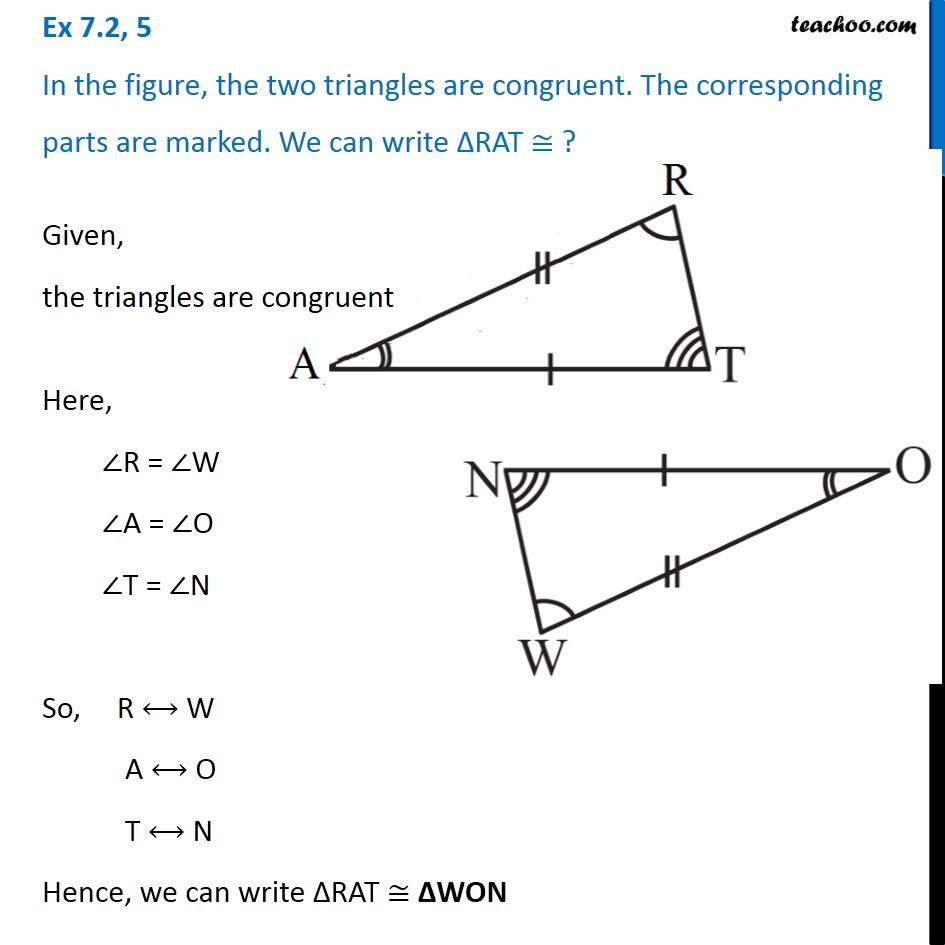Congruency Criteria Mix questions

Congruence of Triangles
Concept wiseLearn in your speed, with individual attention - Teachoo Maths 1-on-1 Class

### Transcript

Question 5 In the figure, the two triangles are congruent. The corresponding parts are marked. We can write ∆RAT ≅ ? Given, the triangles are congruent Here, ∠R = ∠W ∠A = ∠O ∠T = ∠N So, R ⟷ W A ⟷ O T ⟷ N Hence, we can write ∆RAT ≅ ∆WON